## Wednesday, July 26, 2017

### Bibi

(Click on the comic if you can't see the full image.)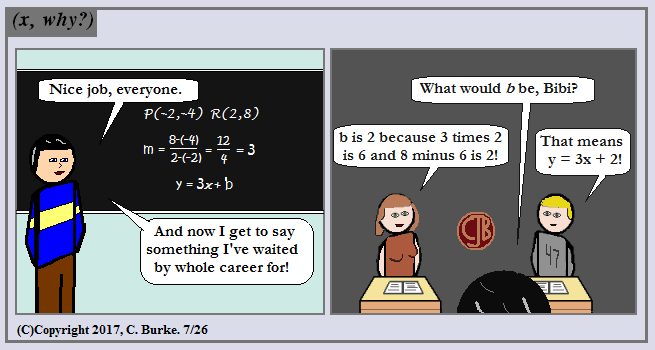There's always that kid that has to jump in to get part of the credit.

I use "Jeopardy" rules -- if you didn't buzz in (raise your hand) and get called on, the person currently answering can steal the answer and get the credit.

And if you think I inserted a girl into the class last week just so I could do this, well, you'd be incorrect, but it certainly helped. I haven't been waiting my whole career for this one, but I did write it in a notepad quite a while ago!

Come back often for more funny math and geeky comics.## Monday, July 24, 2017

### (x, why?) Mini: Shapes of Things To Come

(Click on the comic if you can't see the full image.)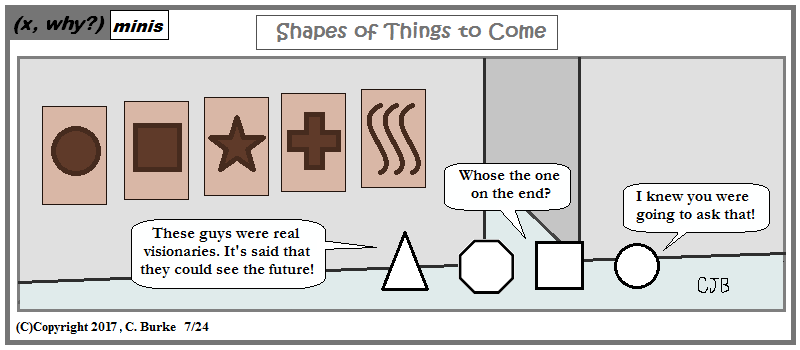But can you predict which shape will be around the corner?

Come back often for more funny math and geeky comics.## Friday, July 21, 2017

### Cosmic Microwaves

(Click on the comic if you can't see the full image.)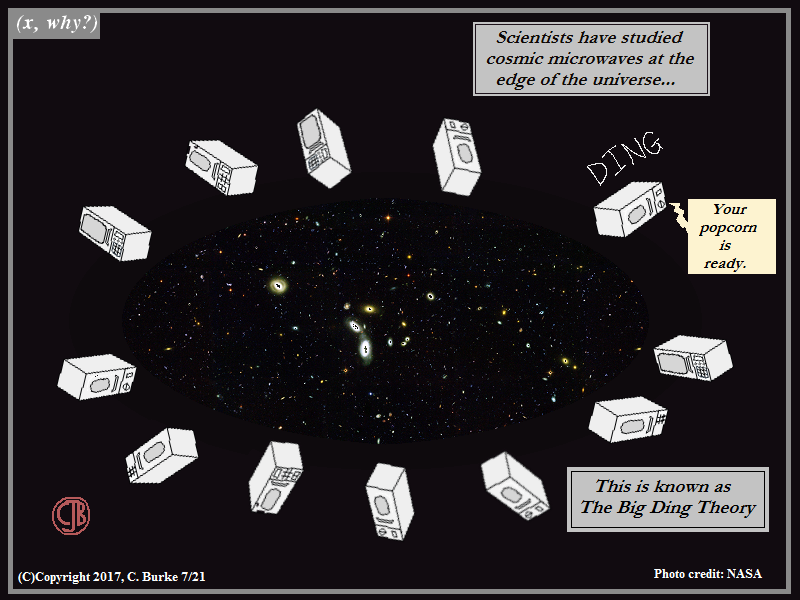What it says on the Tin

If the Microwave was the greatest invention since the invention of popcorn, the Popcorn button was the greatest invention since the microwave!

Come back often for more funny math and geeky comics.## Wednesday, July 19, 2017

### With A Song in My Math

(Click on the comic if you can't see the full image.)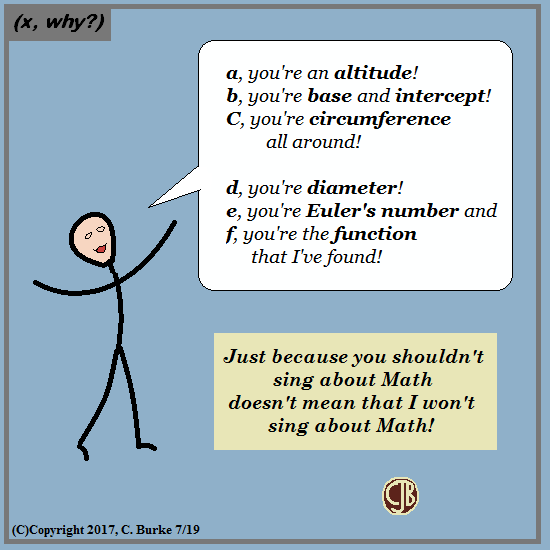I could go on ALL DAY!!!! ... but I won't

Alphabetically speaking, you're OK. No, you're Buddy Kaye!

Come back often for more funny math and geeky comics.## Monday, July 17, 2017

### The Doctor is Still In

(Click on the comic if you can't see the full image.)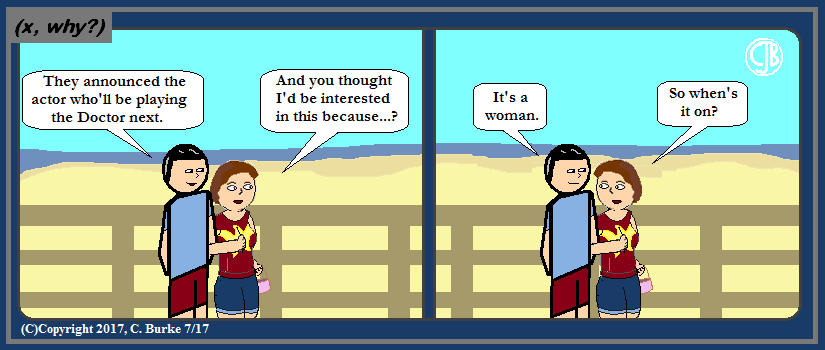When is Who on?

Joke's on Michele: she'll have to sit through a whole Christmas special with two old male Doctors before we get a quick introduction to the new Who.

Oh, and "Spoiler Alert!" if you somehow missed the news.

Come back often for more funny math and geeky comics.## Thursday, July 13, 2017

### Just What the Teacher Inscribed!

(Click on the comic if you can't see the full image.)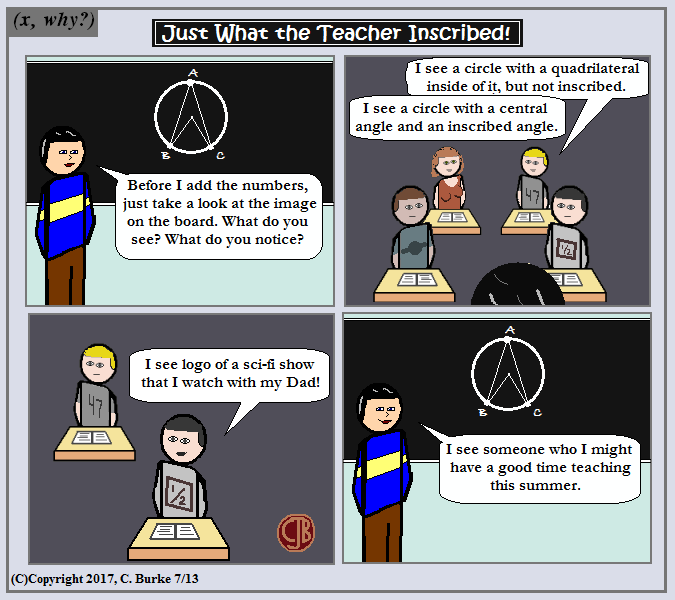I would've put 'Mom and Dad', but I had space limitations. Space: the final frontier.

Plus, from real life, there's a good choice that it's a single parent carrying on the good traditions while the other is poisoning their minds with 'weird stuff'.

Come back often for more funny math and geeky comics.## Tuesday, July 11, 2017

### Don't Think, So?

(Click on the comic if you can't see the full image.)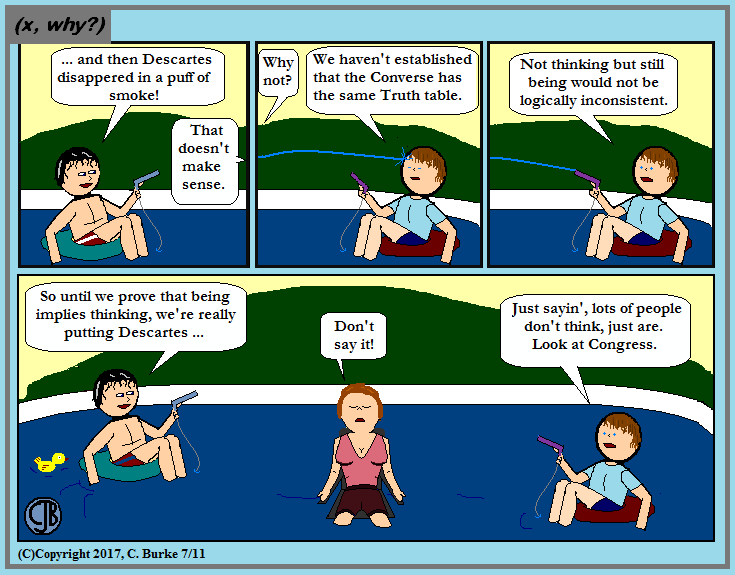I've heard variations enough to think we put Descartes in front of a dead horse, beaten with the same puns.

Of course, if we set the Wayback Machine to 2010, we've tackled Descartes before.

Come back often for more funny math and geeky comics.## Sunday, July 09, 2017

### (x, why?) Mini: Think Proof

(Click on the comic if you can't see the full image.)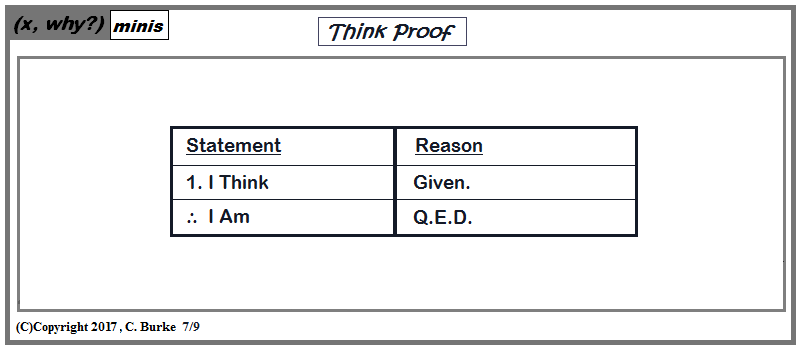Not to be confused with 'Thinkproof' or even 'Thoughtproof', which almost seems to describe some average adults I know.

Come back often for more funny math and geeky comics.## Thursday, July 06, 2017

### Like, Totally Independent

(Click on the comic if you can't see the full image.)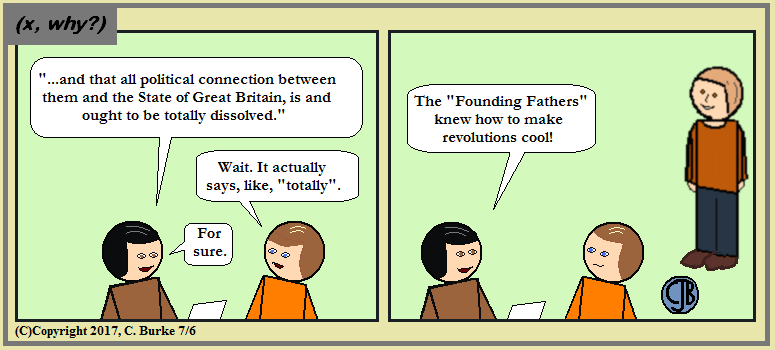Math joke: I can imagine an 'x' separated from the other letters saying he was 'totally independent' from the other variables.
Maybe that can be a future joke(?).

Come back often for more funny math and geeky comics.## Wednesday, July 05, 2017

### June 2017: Common Core Geometry Regents, Part 1

The following are the questions and answers (and commentary) for part of the New York State Algebra Regents exam. If you have any questions or comments (or corrections), please add them in the Comments section.

My apologies for typos, particularly if they are in the questions, because then the answers are subject to change.

Answers to Part II can be found here.

Answers to Part III can be found here.

Answers to Part IV can be found here.

### June 2017, Geometry (Common Core), Part I

1.In the diagram below, triangle ABC = triangle DEF. (image omitted)
Which sequence of transformations maps triangle ABC onto triangle DEF?

(2) a reflection over the y-axis followed by a translation. ABC is flipped over the y-axis, so it is facing the opposite direction. Then it sinks down below the x-axis through a translation.

2.On the set of axes below, the vertices of triangle PQR have coordinates P(-6,7), Q(2,l), and R(-1, -3). (image omitted)
What is the area of triangle PQR?

(3) 25. If you notice, PQ is perpendicular to QR. The slope of PQ is -3/4, and the slope of QR is 4/3. Pythagorean theorem -- as well as knowledge of Pythagorean Triples -- will tell you that PQ = 10 and QR = 5. The area of a triangle is (1/2)(b)(h) = (1/2)(5)(10) = 25.
(You might also remember for several of my comics that the area of a triangle could be written as "one-half a b".)

3.In right triangle ABC, m<C = 90°. If cos B = 5/13, which function also equals 5/13?

(3) sin A. If C is the right angle, then A and B are the acute angles, which are complimentary. The sine of one acute angle will always equal the cosine of the other acute angle, but what is opposite one angle is adjacent to the other.

4.In the diagram below, m<ABC = 268°. (image omitted)
What is the number of degrees in the measure of <ABC?

(4) 46°. The complete circle is 360°. If major arc is ABC = 268, then AC = 360 - 268 = 92. The inscribed angle is half the size of the central angle, so 92 / 2 = 46°.

5.Given triangle MRO shown below, with trapezoid PTRO, MR = 9, MP = 2, and PO = 4. (image omitted)
What is the length of TR?

(4) 6. PO / MO = TR / MR. MO = 6, so 4 / 6 = TR / 9. Cross-multiply and get TR = 6.

6.A line segment is dilated by a scale factor of 2 centered at a point not on the line segment. Which statement regarding the relationship between the given line segment and its image is true?

(3) The line segments are parallel, and the image is twice the length of the given line segment. The image will also be twice as far away from the center than the original line was.

7.Which figure always has exactly four lines of reflection that map the figure onto itself?

(1) Square. Lines through the midpoints of each pair of parallel sides and the two diagonals. An octagon has eight lines: through the four pairs of parallel lines and lines through the opposites vertices.

8.In the diagram below of circle 0, chord D F bisects chord BC at E. (image omitted)
If BC = 12 and FE is 5 more than DE, then FE is

(2) 9. BC is bisected, so BE = CE = 6. The rule for intersecting chords tells us that

(BE)(CE) = (DE)(FE).
(6)(6) = (x)(x + 5)
36 = x2 + 5x

Stop here. If you did this, you're doing too much work for a multiple choice question.

What are the factors of 36?
1, 36; 2, 18; 3, 12; 4, 9; 6, 6.
Which two have a difference of 5? 4 and 9, both of which are choices.
FE is the longer one (it's "5 more"), so the answer is 9.

9.Kelly is completing a proof based on the figure below. (image omitted)
She was given that <A = <EDF, and has already proven AB = DE. Which pair of corresponding parts and triangle congruency method would not prove triangle ABC = triangle DEF?

(2) BC = EF and SAS. SAS requires that the congruent angle be included between the two pairs of congruent sides. BC and EF would give you SSA instead, and that is not allowed (except in the special case of right triangles, where it is HL).

10. In the diagram below, DE divides AB and AC proportionally, m<C = 26°, m<A = 82°, and DF bisects LBDE. (image omitted)
The measure of angle DFB is

(2) 54°. DE divides the two sides proportionally, so DE is parallel to side BC. Angle AED = 26 because it is a corresponding angle. Using the Exterior Angle theorem, we know that <EDB = 82 + 26 = 108. (If you forgot that theorem, you could have found <ADE because a triangle has 180 degrees and then found <EDB because it is supplementary.)

DF bisects <BDE, so <BDF = <EDF = 54. Angle DFB is an alternate interior angle to EDF, so it equals 54°.

You also could have solved it by finding the angles of triangle DBF. Angle B = 180 - 82 - 26 = 72. So <DFB = 180 - 54 - 72 = 54

11.Which set of statements would describe a parallelogram that can always be classified as a rhombus?
I. Diagonals are perpendicular bisectors of each other.
II. Diagonals bisect the angles from which they are drawn.
III. Diagonals form four congruent isosceles right triangles.

Update:
(4) I, II and III. The diagonals of a rhombus will divide it into four congruent isosceles triangles. This can only happen if they are perpendicular to each other, bisect each other and bisect the angles.
Note: There is some important information in this question that could have helped you with the proof in Part IV!

(2) I and III. The diagonals of a rhombus are NOT angle bisectors, unless it's a square.
Note: There is some important information in this question that could have helped you with the proof in Part IV!

I don't know where my head was when I typed the above. Obviously, it's a rectangle where the diagonals only bisect the angles when it is also a square. Just shows you that you should rush yourself, and to always double check. If the angles weren't bisected, how could III by true? Looks like I would not have scored 100 on this test.

12.The equation of a circle is x2 + y2 - 12y + 20 = 0. What are the coordinates of the center and the length of the radius of the circle?

(2) A = 1000(1 + 0.013)2. Interest increases your value, so choices (1) and (3) are right out. The percent 1.3% must be converted to a decimal, which is 0.013. The correct answer is (2). You need to complete the square. Half of -12 is -6, so we need to get to (y - 6)2:

x2 + y2 - 12y + 20 = 0
x2 + y2 - 12y + 36 + 20 = 36
x2 + y2 - 12y + 36 = 16
x2 + (y - 6)2 = 42
This make the center (0, 6) and the radius 4.

13. In the diagram of triangle RST below, m<T = 90°, RS = 65, and ST = 60. (image omitted) What is the measure of <S, to the nearest degree?

(1) 23°. First of all, I hope you realized that RT is the shortest side of the triangle (not because of looks, but because of Pythagorean Triples), so choices (3) and (4) are too big.
We have the adjacent side and the hypotenuse so we need to use cosine to find the angle.
cos S = 60 / 65
S = cos-1 (60 / 65) = 22.62..., which rounds to 23.

14. Triangle A'B'C' is the image of triangle ABC after a dilation followed by a translation. Which statement(s) would always be true with respect to this sequence of transformations?
I. Triangle ABC = triangle A'B'C'
II. Triangle ABC ~ triangle A'B'C'
III. AB || A'B'
IV. AA'= BB'

As a result of discrepancies in the wording, Questions 14 does not have one clear and correct answer.
Either (1) II, only or (3) II and III were accepted.
I don't know what the discrepancy might be that would make someone not think that AB was not parallel to A'B', unless there were printing errors in some books that left out one of the accent marks.

A dilation will preserve the shape of the original object, so the original and the image must be similar.
The center point is not given, but regardless, the orientation will not change, so the slopes of the sides will not change, so the sides will be parallel.
Update: (3) is an acceptable answer because it is possible, since exact values were not given, that after the translation, the dilation could cause AB and A'B' to become part of the same line, and not parallel. (Thank you to those who commented and emailed me.)

15. Line segment RW has endpoints R(-4,5) and W(6,20). Point P is on RW such that RP:PW is 2:3. What are the coordinates of point P?

(2) (0,11). Add 2 + 3 = 5, so RP is 2/5 the length of RW. Find difference of the x-values and y-values of RW and multiply by 2/5.
2/5(6 - (-4)) = 2/5(10) = 4
2/5(20 - 5) = 2/5(15) = 6
Add +4,+6 to point R(-4, 6). P(-4 + 4, 5 + 6) = P(0, 11).

16. The pyramid shown below has a square base, a height of 7, and a volume of 84. (image omitted)
What is the length of the side of the base?

(1) 6. Volume = (1/3)(Area of Base)(height)
84 = (1/3)(B)(7)
252 = 7B
36 = Area of the Base.
The base is a square, then the length of one side is the square root of 36, which is 6

17.In the diagram below of triangle MNO, <M and <O are bisected by MS and OR, respectively. Segments MS and OR intersect at T, and m<N = 40°. (image omitted)
If m<TMR = 28°, the measure of angle OTS is

(4) 70°. Again, we can use the Exterior Angle Theorem to figure this out.
TMR = 28, but since MS bisects <M, <TMO is also 28, and <OMN = 56°. Since <N = 40°, <MON = 180 - 40 - 56 = 84°. Since OR bisects <MON, then <MOT and <SOT are each 42°.
Look at triangle MOT. If <TMO = 28 and <MOT = 42, then <OTS = 28 + 42 = 70°.

18.In the diagram below, right triangle ABC has legs whose lengths are 4 and 6. (image omitted)
What is the volume of the three-dimensional object formed by continuously rotating the right triangle around AB?

(1) 32 pi. First, rotating the triangle creates a cone. The Volume of a cone is (1/3)(Area of the Base)(height). The area of the base is (pi)(r)2, where the radius is 4. The height is 6 because AB is the axis the triangle is rotated about.
V = (1/3)(pi)(4)2(6) = (1/3)(16)(pi)(6) = 32 pi.

If you look at the incorrect answers: (2) assumes you used 6 as the radius (rotating about AC), (3) assumes you forgot the 1/3, and (4) assumes that you made both mistakes.

19.What is an equation of a line that is perpendicular to the line whose equation is 2y = 3x - 10 and passes through (-6,1)?

(2) y = -2/3 x - 3. The slope of the line in the question is 3/2. The slope of a perpendicular line must be -2/3 because it is the inverse reciprocal, so choices (3) and (4) are out.
Substitute -6 for x in choices (1) and (2).
(1) y = -2/3 (-6) - 5 = 4 - 5 = -1. No good. We don't want (-6, -1)
(2) y = -2/3 (-6) - 3 = 4 - 3 = 1. Check.

20. In quadrilateral BLUE shown below, BE ~ UL. (image omitted)
Which information would be sufficient to prove quadrilateral BLUE is a parallelogram?

(2) LU || BE. If a pair of line segments in a quadrilateral are both parallel and congruent, then the quadrilateral in a parallelogram. This information could be used in the proof in Part IV.
Note that choices (3) and (4) only show that three sides are congruent, not four. That could be a trapezoid.

21. A ladder 20 feet long leans against a building, forming an angle of 71° with the level ground. To the nearest foot, how high up the wall of the building does the ladder touch the building?

(4) 19 You know the length of the ladder, which is the hypotenuse of the right triangle formed by the ladder, the ground and the wall. The wall is opposite the 71° angle. So you need to use sine to find the answer.

So sin 71° = x / 20
x = 20 sin 71° = 18.91 = 19 feet.

22.In the two distinct acute triangles ABC and DEF, <B = <E. Triangles ABC and DEF are congruent when there is a sequence of rigid motions that maps

As a result of discrepancies in the wording, Questions 22 does not have one clear and correct answer. As a result, all choices were accepted.

23.A fabricator is hired to make a 27-foot-long solid metal railing for the stairs at the local library. The railing is modeled by the diagram below. The railing is 2.5 inches high and 2.5 inches wide and is comprised of a rectangular prism and a half-cylinder. (image omitted)
How much metal, to the nearest cubic inch, will the railing contain?

(1) 151. A reminder: there is no simple one-step formula that will give you this answer!
First you have to differentiate how much of the 2.5 inch height is part of the rectangular prism and what is part of the half-cylinder. The diameter of the half-cylinder is 2.5 in, which means that the radius is 1.25 in. Therefore the height of the prism is 1.25.

So the Volume of the railing = the Volume of the prism + the Volume of the half-cylinder
V = L * W * H + (1/2) (pi) (r)2 h
V = (2.5)(1.25)(27) + (1/2)(pi)(1.25)2(27)
V = 84.375 + 66.268..
V = 150.643... = 151

CORRECTION: I usually look for such things, but I skipped over converting the feet into inches. That's what I get for not checking my work against the answer key when it became available! (And thanks for pointing this out.)

(3) 1808. A reminder: there is no simple one-step formula that will give you this answer!
First you have to differentiate how much of the 2.5 inch height is part of the rectangular prism and what is part of the half-cylinder. The diameter of the half-cylinder is 2.5 in, which means that the radius is 1.25 in. Therefore the height of the prism is 1.25.

So the Volume of the railing = the Volume of the prism + the Volume of the half-cylinder
V = L * W * H + (1/2) (pi) (r)2 h
(27) * (12) = 324
V = (2.5)(1.25)(324) + (1/2)(pi)(1.25)2(324)
V = 1012.5 + 795.215..
V = 1807.7 = 1808

24.In the diagram below, AC = 7.2 and CE = 2.4. (image omitted)
Which statement is not sufficient to prove triangle ABC ~ triangle EDC?

(2) DE = 2.7 and AB = 8.1. In Choice (1), the parallel lines create alternate interior angles that are congruent, so the triangles are similar by AA (or AAA). The other three choices are show ratios that are 3:1, so the numbers themselves are not important. What is important is which sides are given. Keep in mind that there are vertical angles, so we do have one pair of congruent angles. So the other choices need to give us SAS or SSS. Choice (2) gives us SSA, which isn't allowed.

Update:
As noted in the comments below, this question, as written, has NO answer. It has a "best" answer, which could be argued only means that it's the "least wrong", which is still wrong. The Regents Board has stated that they are not rescoring the exams. Actually, I don't blame them -- the test is bad enough without making it worse. Allow me to explain my point of view:

Two other questions on this test had problems. One was invalidated because there was no positive correct answer, so everyone received credit. In another case, they accepted two answers for which credit was given. The passing grade for this exam is already artificially low. I would prefer a less confusing exam (I wouldn't say "less rigorous", but maybe that, too) with a higher threshold for passing, and a curve which doesn't punish the high-achievers.

I won't try to suggest that the Regents exam models real life any more than the SATs do. But test taking itself is a skill, and sometimes you do have to find the "best" solution, or even the "least worst", and not the "right" or the "one true" answer.

Now, I could go either way, depending upon the question. If the wording is so ambiguous as to leave a student guessing between two different choices depending on how you read something, that's a no-brainer: toss it. And if something is left out so that there is no positive answer, that should be immediately thrown out as well. Question 24, on the other hand, is a little different. First of all, you aren't looking for a positive answer at all; you want a negative answer. Which one is NOT sufficient, which means that you should be able to show that at least three of them are. Keeping in mind that this is a multiple-choice question, students are not expected to show any work at all, though they are encouraged to do so in the margins of the book. They are NOT expected to write two-page proofs in the margins, and most would not.

Now, let me stop and say this: I applaud any student that has the determination to write a two-page proof in the margins.

If you were to answer this Geometry question about similarity, the first step would be use the Laws of Similarity and Congruence. These are quick to use and quickly eliminate three of the four choices. At this point, one might select the fourth option (Choice 2) as being the correct response. At the very least, it is the one the Regents were looking for. (Yes, I hate when teachers make you guess the solution that they are looking for, whether or not it is the one that makes the most sense -- However, in this case, it is the one that makes the most sense.)

I was troubled by this question when I first saw it, because it gave exact numbers. I originally thought it would be a calculation problem, but the numbers proved little use in the methods shown above. That doesn't mean that they couldn't be useful for different methods.

In particular, you could use the Law of Sines to compare the ratios and find the angle between the sides. Given that there is a pair of vertical angles in the diagram, that is enough to prove similarity by AA. Instead of a logic-based argument, you could do a calculation-based argument.

But here's the thing: asking a student to do that in an Open-Ended problem would be difficult enough that I wouldn't even expect to find it in Part II. That would be a Part III question, at least, worth a minimum of 4 points. No one would expect any student, particularly one who "reviewed for the test" -- sadly, a necessary skill these days -- to do any of that on a multiple-choice question. Again, to those of you who go these extra lengths: More Power to You! But, sadly, using the Law of Sines in this situation probably wouldn't occur to most students even if, a) they were taught the concept properly, and b) they could execute it to its conclusion.

Finally, this isn't a case of confusion, but one of omission. There actually is a quick fix for this problem. Had the question stated "through the rules for Similarity and Congruency" (or something similar), their answer would be valid, but I suspect that such an addition would be deemed as giving too much away.

Oddly, it also could have been corrected had they switched the numbers around so that AB and DE were not the longest sides, which is an important part of Benjamin Catalfo's proof, but I doubt that they expected anyone to go this far on a multiple-choice question. Hopefully, they are now thus informed and won't make the same mistake twice. Likewise, I hope that Mr. Catalfo, upon finding no correct answer, was wise enough to choose the one that they were looking for. Sadly, sometimes this is how math -- and education, in general -- models the "real world".

End of Part I

How did you do?
Comments, questions, corrections and concerns are all welcome.
Typos happen.

### I also write Fiction!

Check out In A Flash 2020, by Christopher J. Burke for 20 great flash fiction stories, perfectly sized for your train rides.
Available in softcover or ebook at Amazon.

If you enjoy it, please consider leaving a rating or review on Amazon or on Good Reads.

Thank you.## Tuesday, July 04, 2017

### Happy Fourth of July 2017!

(Click on the comic if you can't see the full image.)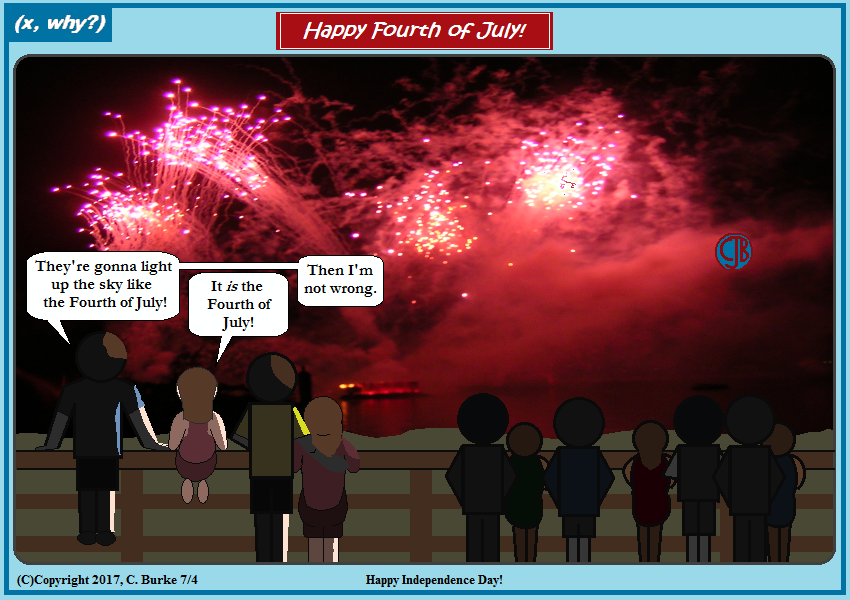Can't be more like it than actually being it.

Mike's wife is thinking, "No one better be taking pictures from behind me."
I know this from experience.

I probably should add that I took that photo during a family vacation some years ago, so I'm the copyright holder. It is not in public domain.

Come back often for more funny math and geeky comics.